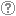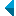# Iskanje po repozitorijuIskalni niz: išči po NaslovAvtorOpisKljučne besedePolno besediloLeto izida INALIIN NE išči po NaslovAvtorOpisKljučne besedePolno besediloLeto izida INALIIN NE išči po NaslovAvtorOpisKljučne besedePolno besediloLeto izida INALIIN NE išči po NaslovAvtorOpisKljučne besedePolno besediloLeto izida Vrsta gradiva: Vse vrste gradiv Habilitacijsko delo (m4) Višješolska diplomska naloga (m6) Specialistično delo (m3) Diplomsko delo * (dip) Magistrsko delo * (mag) Doktorska disertacija * (dok) Raziskovalni podatki ali korpus (data) * po starem in bolonjskem študiju Jezik: Vsi jezikiSlovenski jezikAngleški jezikNemški jezikHrvaški jezikSrbski jezikBolgarski jezikBosanski jezikČeški jezikFinski jezikFrancoski jezikItalijanski jezikJaponski jezikLitvanski jezikMadžarski jezikNemški jezik (Avstrija)Norveški jezikPoljski jezikRuski jezikSlovaški jezikSrbski jezik (cirilica)Španski jezikŠvedski jezikTurški jezikNeznan jezik Išči po: RUP    FAMNIT - Fakulteta za matematiko, naravoslovje in informacijske tehnologije    FHŠ - Fakulteta za humanistične študije    FM - Fakulteta za management    FTŠ Turistica - Fakulteta za turistične študije - Turistica    FVZ - Fakulteta za vede o zdravju    IAM - Inštitut Andrej Marušič    PEF - Pedagoška fakulteta    UPR - Univerza na Primorskem    ZUP - Založba Univerze na PrimorskemCOBISS    Fakulteta za humanistične študije, Koper    Fakulteta za management Koper in Pedagoška fakulteta Koper    Fakulteta za vede o zdravju, Izola    Knjižnica za tehniko, medicino in naravoslovje, Koper    Turistica, Portorož    Znanstveno-raziskovalno središče Koper Opcije: Prikaži samo zadetke s polnim besedilom Ponastavi

 1 - 10 / 11121.Identities with generalized skew derivations on Lie idealsAjda Fošner, Vincenzo De Filippis, Feng Wei, 2013, izvirni znanstveni članekOpis: Let ▫$m, n$▫ be two nonzero fixed positive integers, ▫$R$▫ a 2-torsion free prime ring with the right Martindale quotient ring ▫$Q$▫, ▫$L$▫ a non-central Lie ideal of ▫$R$▫, and ▫$\delta$▫ a derivation of ▫$R$▫. Suppose that ▫$\alpha$▫ is an automorphism of ▫$R$▫, ▫$D$▫ a skew derivation of ▫$R$▫ with the associated automorphism ▫$\alpha$▫, and ▫$F$▫ a generalized skew derivation of ▫$R$▫ with the associated skew derivation ▫$D$▫. If ▫$$F(x^{m+n}) = F(x^m)x^n + x^m \delta (x^n)$$▫ is a polynomial identity for ▫$L$▫, then either ▫$R$▫ satisfies the standard polynomial identity ▫$s_4(x_1, x_2, x_3, x_4)$▫ of degree 4, or ▫$F$▫ is a generalized derivation of ▫$R$▫ and ▫$\delta = D$▫. Furthermore, in the latter case one of the following statements holds: (1) ▫$D = \delta = 0$▫ and there exists ▫$a \in Q$▫ such that ▫$F(x) = ax$▫ for all ▫$x \in R$▫; (2) ▫$\alpha$▫ is the identical mapping of ▫$R$▫.Najdeno v: osebiKljučne besede: mathematics, algebra, polynomial identity, generalized skew derivation, prime ringObjavljeno: 15.10.2013; Ogledov: 3139; Prenosov: 138Polno besedilo (0,00 KB) 2.On 2-fold covers of graphsKlavdija Kutnar, Yan-Quan Feng, Aleksander Malnič, Dragan Marušič, 2008, izvirni znanstveni članekOpis: A regular covering projection ▫$\wp : \widetilde{X} \to X$▫ of connected graphs is ▫$G$▫-admissible if ▫$G$▫ lifts along ▫$\wp$▫. Denote by ▫$\tilde{G}$▫ the lifted group, and let CT▫$(\wp)$▫ be the group of covering transformations. The projection is called ▫$G$▫-split whenever the extension ▫{$\mathrm{CT}}(\wp) \to \tilde{G} \to G$▫ splits. In this paper, split 2-covers are considered, with a particular emphasis given to cubic symmetric graphs. Supposing that ▫$G$▫ is transitive on ▫$X$▫, a ▫$G$▫-split cover is said to be ▫$G$▫-split-transitive if all complements ▫$\tilde{G} \cong G$▫ of CT▫$(\wp)$▫ within ▫$\tilde{G}$▫ are transitive on ▫$\widetilde{X}$▫; it is said to be ▫$G$▫-split-sectional whenever for each complement ▫$\tilde{G}$▫ there exists a ▫$\tilde{G}$▫-invariant section of ▫$\wp$▫; and it is called ▫$G$▫-split-mixed otherwise. It is shown, when ▫$G$▫ is an arc-transitive group, split-sectional and split-mixed 2-covers lead to canonical double covers. Split-transitive covers, however, are considerably more difficult to analyze. For cubic symmetric graphs split 2-cover are necessarily canonical double covers (that is, no ▫$G$▫-split-transitive 2-covers exist) when ▫$G$▫ is 1-regular or 4-regular. In all other cases, that is, if ▫$G$▫ is ▫$s$▫-regular, ▫$s=2,3$▫ or ▫$5$▫, a necessary and sufficient condition for the existence of a transitive complement ▫$\tilde{G}$▫ is given, and moreover, an infinite family of split-transitive 2-covers based on the alternating groups of the form ▫$A_{12k+10}$▫ is constructed. Finally, chains of consecutive 2-covers, along which an arc-transitive group ▫$G$▫ has successive lifts, are also considered. It is proved that in such a chain, at most two projections can be split. Further, it is shown that, in the context of cubic symmetric graphs, if exactly two of them are split, then one is split-transitive and the other one is either split-sectional or split-mixed.Najdeno v: osebiKljučne besede: graph theory, graphs, cubic graphs, symmetric graphs, ▫$s$▫-regular group, regular covering projectionObjavljeno: 15.10.2013; Ogledov: 2673; Prenosov: 31Polno besedilo (0,00 KB) 3.Elementary abelian groups of rank 5 are DCI-groupsIstván Kovács, Yan-Quan Feng, 2018, objavljeni povzetek znanstvenega prispevka na konferenci (vabljeno predavanje)Najdeno v: osebiKljučne besede: elementary abelian group, rank, DCI-groupObjavljeno: 07.02.2018; Ogledov: 1676; Prenosov: 73Polno besedilo (0,00 KB) 4.Quasi-semiregular automorphisms of cubic and tetravalent arc-transitive graphsKlavdija Kutnar, Ademir Hujdurović, Dragan Marušič, Yan-Quan Feng, István Kovács, 2018, prispevek na konferenci brez natisaNajdeno v: osebiKljučne besede: quasi-semiregular automorphism, cubic graph, tetravalent graph, arc-transitive graphObjavljeno: 06.12.2018; Ogledov: 1362; Prenosov: 123Polno besedilo (0,00 KB) 5.Quasi-semiregular automorphisms of cubic and tetravalent arc-transitive graphsKlavdija Kutnar, István Kovács, Dragan Marušič, Ademir Hujdurović, Yan-Quan Feng, 2019, izvirni znanstveni članekNajdeno v: osebiKljučne besede: cubic graph, tetravalent graph, arc-transitive, quasi-semiregular automorphismObjavljeno: 14.03.2019; Ogledov: 1049; Prenosov: 248Polno besedilo (0,00 KB) 6.On groups all of whose Haar graphs are Cayley graphsIstván Kovács, Da-Wei Yang, Yan-Quan Feng, 2019, izvirni znanstveni članekNajdeno v: osebiKljučne besede: graph automorphism, Cayley graph, Haar graphObjavljeno: 28.06.2019; Ogledov: 1299; Prenosov: 327Polno besedilo (0,00 KB)Gradivo ima več datotek! Več... 7.On cubic symmetric non-Cayley graphs with solvable automorphism groupsDa-Wei Yang, Dragan Marušič, Klavdija Kutnar, Yan-Quan Feng, 2019, izvirni znanstveni članekNajdeno v: osebiKljučne besede: symmetric graph, non-Cayley graph, regular coverObjavljeno: 18.11.2019; Ogledov: 1378; Prenosov: 90Polno besedilo (0,00 KB) 8.Existence of non-Cayley Haar graphsDa-Wei Yang, Jie Wang, István Kovács, Yan-Quan Feng, 2020, izvirni znanstveni članekNajdeno v: osebiKljučne besede: graph, Cayley graph, Haar graphObjavljeno: 17.06.2020; Ogledov: 701; Prenosov: 93Polno besedilo (0,00 KB) 9.On finite groups with prescribed two-generator subgroups and integral Cayley graphsYan-Quan Feng, István Kovács, 2021, izvirni znanstveni članekNajdeno v: osebiKljučne besede: 2-group, Frobenius group, special groupObjavljeno: 06.05.2021; Ogledov: 322; Prenosov: 25Polno besedilo (0,00 KB) 10.The signless Laplacian spectral radius of bounded degree graphs on surfacesLihua Feng, Guihai Yu, Aleksandar Ilić, Dragan Stevanović, 2015, izvirni znanstveni članekNajdeno v: osebiKljučne besede: signless Laplacian matrix, spectral radius, Euler genus, outerplanar graph, Halin graphObjavljeno: 03.04.2017; Ogledov: 1796; Prenosov: 146Polno besedilo (0,00 KB)
Iskanje izvedeno v 0 sek.
Na vrh